# Chapter 17 The Foreign Exchange Market Foreign Exchange

• Slides: 18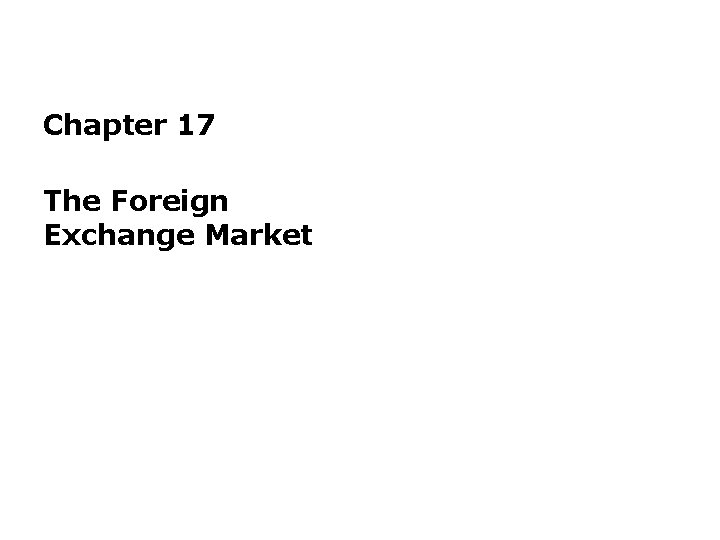Chapter 17 The Foreign Exchange Market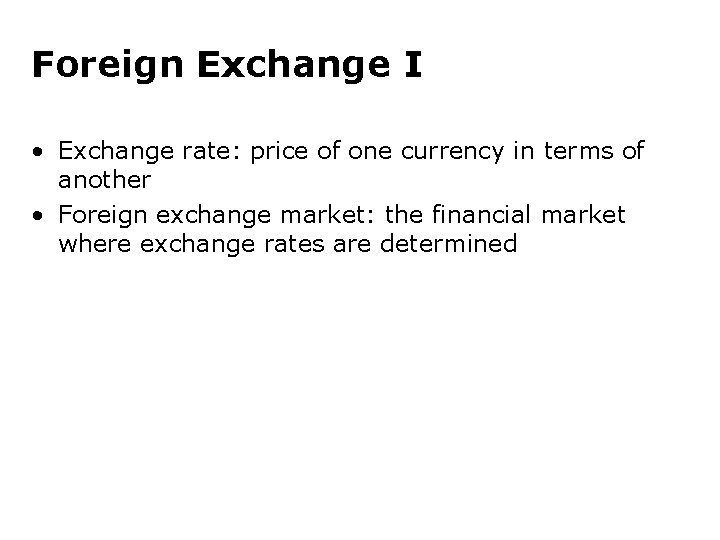Foreign Exchange I • Exchange rate: price of one currency in terms of another • Foreign exchange market: the financial market where exchange rates are determinedForeign Exchange II • Appreciation: a currency rises in value relative to another currency • Depreciation: a currency falls in value relative to another currency • When a country’s currency appreciates, the country’s goods abroad become more expensive and foreign goods in that country become less expensive and vice versa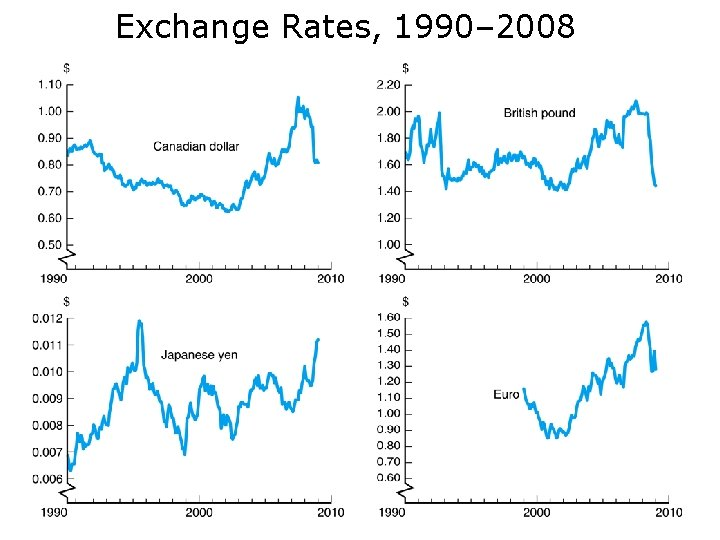Exchange Rates, 1990– 2008Exchange Rates in the Long Run • Law of one price • Theory of Purchasing Power Parity assumptions: – All goods are identical in both countries – Trade barriers and transportation costs are low – Many goods and services are not traded across borders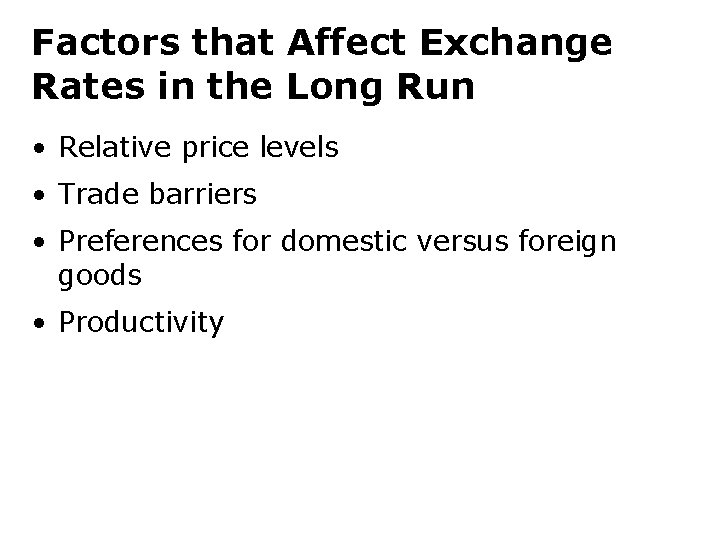Factors that Affect Exchange Rates in the Long Run • Relative price levels • Trade barriers • Preferences for domestic versus foreign goods • ProductivityPurchasing Power Parity, United Kingdom/United States, 1973– 2008 (Index: March 1973 = 100)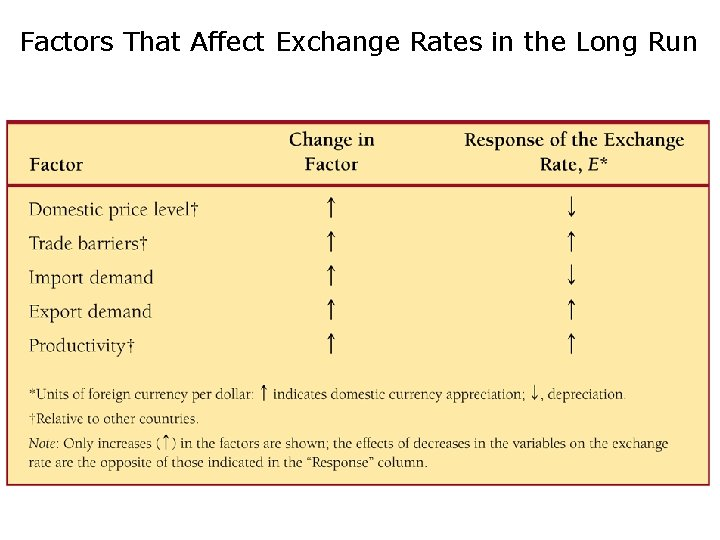Factors That Affect Exchange Rates in the Long Run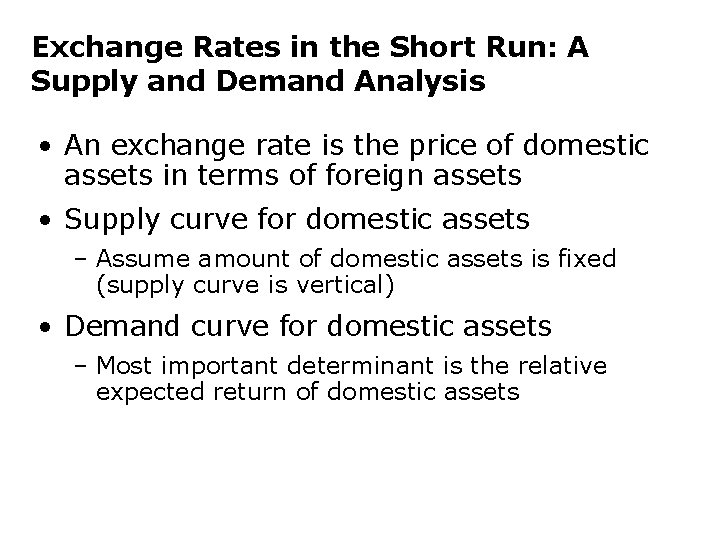Exchange Rates in the Short Run: A Supply and Demand Analysis • An exchange rate is the price of domestic assets in terms of foreign assets • Supply curve for domestic assets – Assume amount of domestic assets is fixed (supply curve is vertical) • Demand curve for domestic assets – Most important determinant is the relative expected return of domestic assets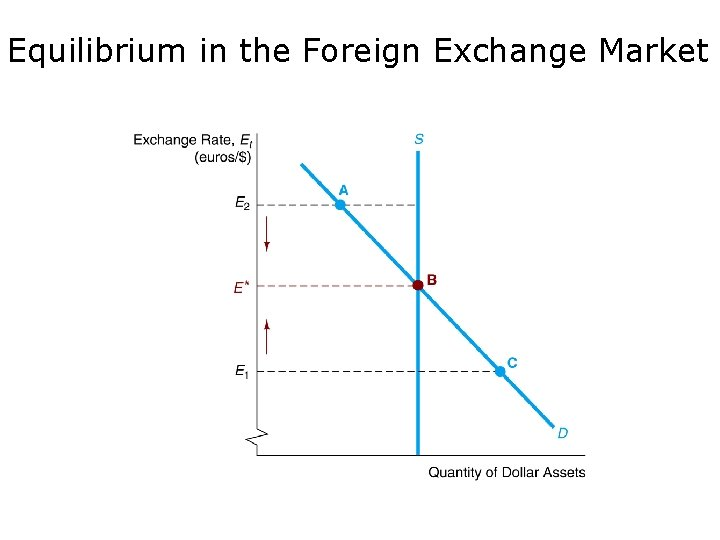Equilibrium in the Foreign Exchange MarketExplaining Changes in Exchange Rates • Shifts in the demand for domestic assets – Domestic interest rate – Foreign interest rate – Expected future exchange rate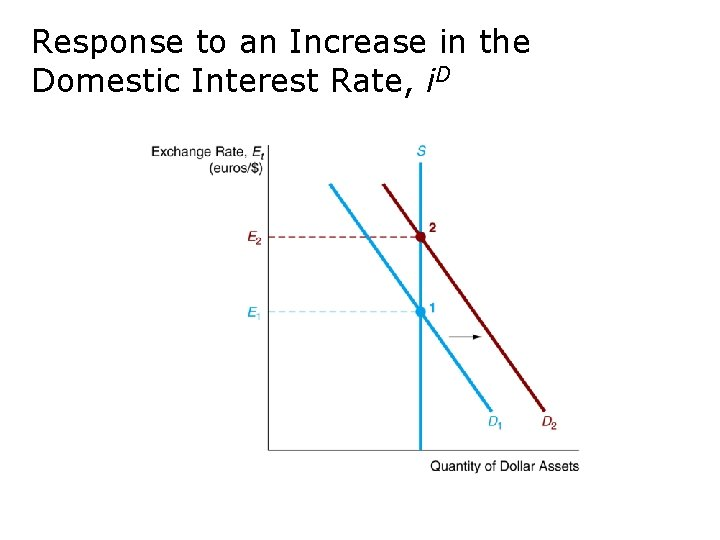Response to an Increase in the Domestic Interest Rate, i. DResponse to an Increase in the Foreign Interest Rate, i. F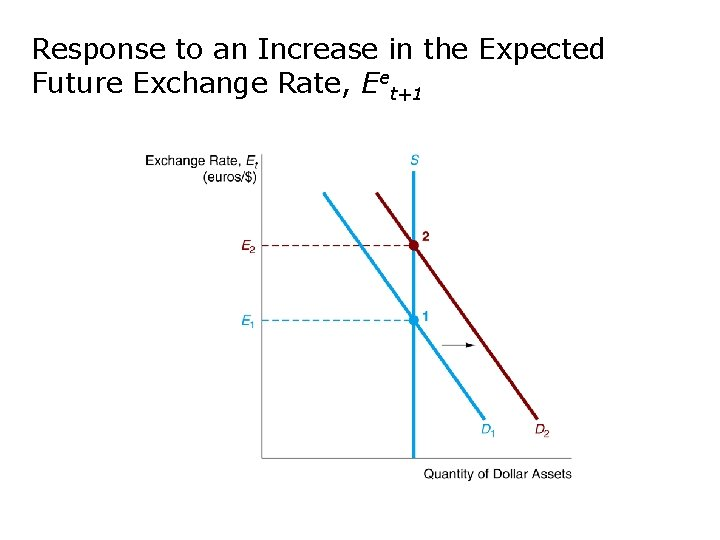Response to an Increase in the Expected Future Exchange Rate, Eet+1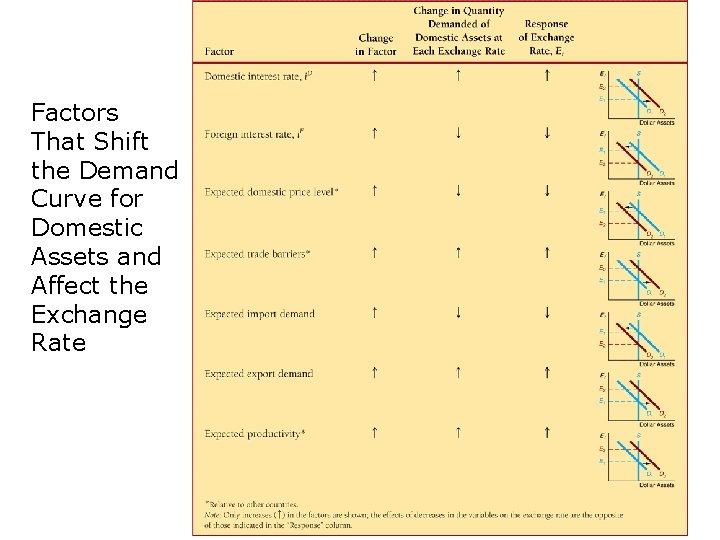Factors That Shift the Demand Curve for Domestic Assets and Affect the Exchange Rate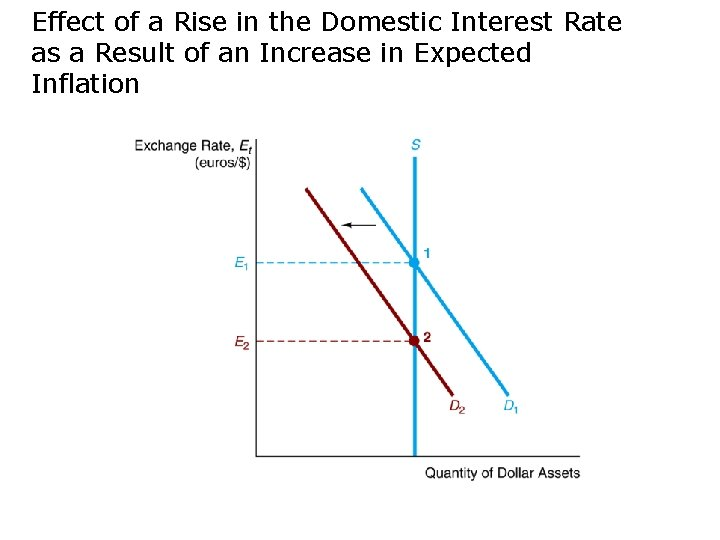Effect of a Rise in the Domestic Interest Rate as a Result of an Increase in Expected Inflation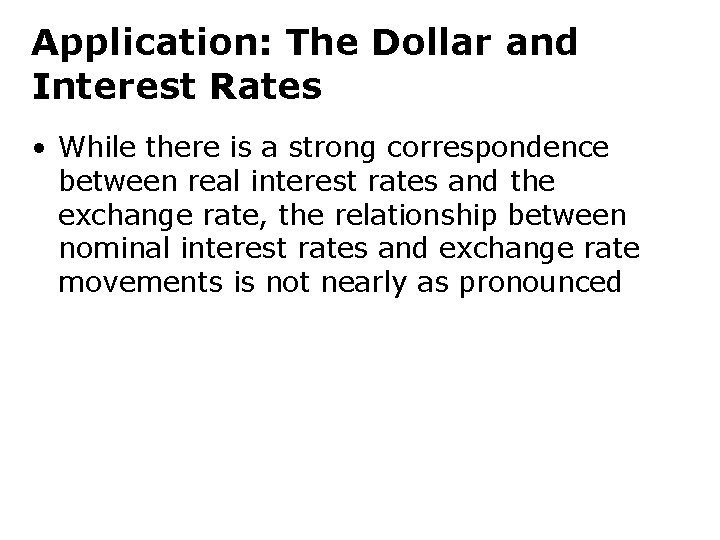Application: The Dollar and Interest Rates • While there is a strong correspondence between real interest rates and the exchange rate, the relationship between nominal interest rates and exchange rate movements is not nearly as pronounced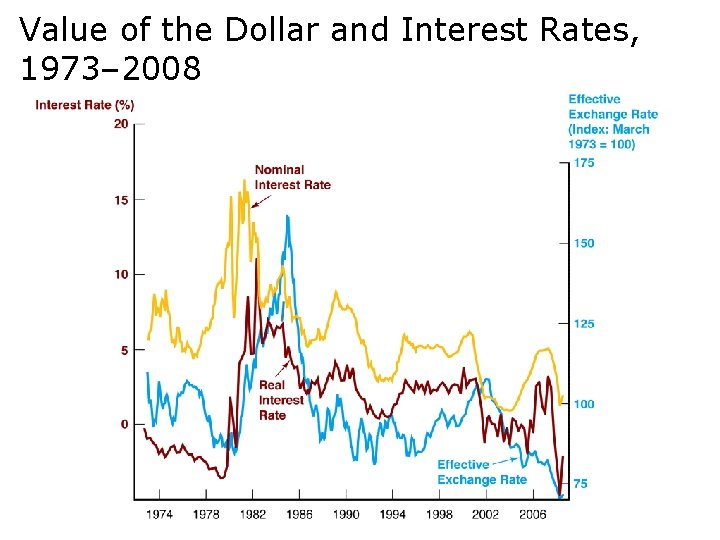Value of the Dollar and Interest Rates, 1973– 2008